# Study Guide

## Elementary Education (Grades 1–6)elementary education left paren grades 1 to 6 right paren Sample Multiple-Choice Questions

Recommendation for individuals using a screenreader: please set your punctuation settings to "most."

### Field 198: Mathematics (Grades 1–6)field 198: mathematics left paren grades 1 to 6 right paren

Each multiple-choice question has four answer choices. Read each question and its answer choices carefully and choose the ONE best answer.

During the test you should try to answer all questions. Even if you are unsure of an answer, it is better to guess than not to answer a question at all. You will NOT be penalized for choosing an incorrect response.

#### Objective 0001 Apply knowledge of college algebra and statistics.

1. Which of the following graphs could represent the polynomial equation  y equals the quantity x plus y times the quantity x plus 5 times the quantity x minus 2?

Each response is a Cartesian graph with a single data line. The x axis is labeled from negative 10 to 10 in increments of 1, and the y axis is labeled from negative 60 to 50 in increments of 10.

1.the data line begins in the lower left quadrant and curves almost vertically up to 30 on the y axis, where it reverses and curves down through 3 on the x axis. at the level of about negative 5 on the y axis, it reverses direction to curve up through 5 on the x axis and continue almost vertically up through the upper right quadrant.

2.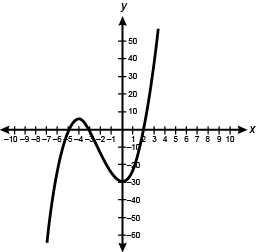the data line begins in the lower left quadrant and curves almost vertically up through negative 5 on the x axis. at the level of about 5 on the y axis, it reverses direction to curve down through negative 3 on the x axis. at negative 30 on the y axis, it reverses direction to curve up through 2 on the x axis and continue almost vertically up through the upper right quadrant.

3.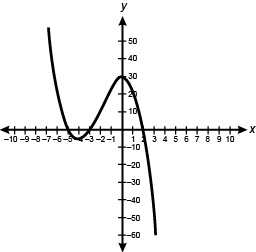the data line begins in the upper left quadrant and curves almost vertically down through negative 5 on the x axis. at the level of about negative 5 on the y axis, it reverses direction to curve up through negative 3 on the x axis. at 30 on the y axis, it reverses direction to curve down through 2 on the x axis and continue almost vertically down through the lower right quadrant.

4.the data line begins in the upper left quadrant and curves almost vertically down through negative 2 on the x axis to negative 30 on the y axis, where it reverses and curves up through 3 on the x axis. at the level of about 5 on the y axis, it reverses direction to curve down through 5 on the x axis and continue almost vertically down through the lower right quadrant.

Answer and Rationale. Enter to expand or collapse. Answer expanded
Correct Response: B.
The equation y equals left paren x plus 3 right paren left paren x plus 5 right paren left paren x minus 2 right paren is the factored form of a third-degree polynomial function. The roots of a polynomial equation are the values of x that will make the value of y equal zero and show up on the graph as the x-intercepts. One method of solving for the roots of a polynomial equation is to factor the equation and then set each factor equal to zero. In the given question, the equation is already factored, and setting the individual factors equal to zero results in x plus 3 equals 0, so  x equals negative 3;   x plus 5 equals 0  so  x equals negative 5 semi  and  x minus 2 equals 0  so  x equals 2  The three roots are  x equals negative 3,   negative 5,  or 2, so the graph will cross the x-axis at the points  left paren negative 3 comma 0 right paren   left paren negative 5 comma 0 right paren  and  left paren 2 comma 0 right paren  While each graph represents a third-degree polynomial function, the only graph that has the correct x-intercepts is the one shown in response B.

#### Objective 0001 Apply knowledge of college algebra and statistics.

2. A community health center conducted a survey to determine how long clerical staff had been in their current positions. The results reported a mean of 28 months, a median of 23 months, and an interquartile range of 14 months. Which of the following box plots could represent these data?

Each response is a box plot with tick marks in increments of 2, labeled from 0 to 50 in increments of 10.

1.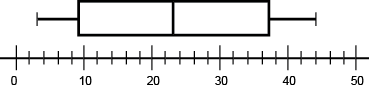The minimum line is at 3, the left edge of the box is at 9, the line inside the box is at 23, the right edge of the box is at 37, and the maximum line is at 44.

2.The minimum line is at 8, the left edge of the box is at 16, the line inside the box is at 28, the right edge of the box is at 42, and the maximum line is at 48.

3.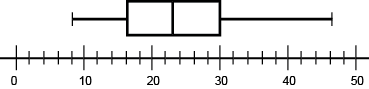The minimum line is at 8, the left edge of the box is at 16, the line inside the box is at 23, the right edge of the box is at 30, and the maximum line is at 46.

4.The minimum line is at 14, the left edge of the box is at 21, the line inside the box is at 28, the right edge of the box is at 35, and the maximum line is at 42.

Answer and Rationale. Enter to expand or collapse. Answer expanded
Correct Response: C.
A box plot displays the median, upper quartile, lower quartile, minimum, and maximum as parts of the graph. The median, given to be 23 months, is true for either of the box plots shown in response A or C, as indicated by the vertical line displayed inside the box of each plot corresponding to the value of 23. To find the interquartile range, find the difference between the upper quartile and the lower quartile. The plot in response C has an upper quartile of 30, as indicated by the vertical line displayed on the right side of the box corresponding to the value of 30, and a lower quartile of 16, displayed as a vertical line on the left side of the box corresponding to the value of 16. The difference between these two values is  30 minus 16,  or 14. The box plot that could represent this data set is shown in response C.

#### Objective 0002 Apply knowledge of the mathematics curriculum and strategies for teaching counting and cardinality, numbers, and operations in base ten.

3. Use the problem and student work below to answer the question that follows.

Problem:
A school is planning an assembly for the last day of the school year. There are 100 fourth graders in the school. The principal has arranged the chairs in the auditorium into rows of eight. How many rows does the principal need to save to seat all the fourth graders?

Student work:

100 divided by 8 equals blank 12 times 8 equals 96 100 minus 96 equals 4 The principal has to save 12 rows.

Which of the following statements is the best evaluation of the student's work?

1. The student's answer is incorrect and the work shows a misunderstanding of how to interpret the remainder in a real-world problem.
2. The student's answer is incorrect and the work shows a misunderstanding of how to perform the common division algorithm.
3. The student's answer is correct and the work shows an understanding of the relationship between division and multiplication.
4. The student's answer is correct and the work shows an understanding of how to model division problems using repeated subtraction.
Answer and Rationale. Enter to expand or collapse. Answer expanded
Correct Response: A.
This problem requires the candidate to divide the total number of fourth graders by the number of chairs per row. All the fourth graders need to be seated so any remainder will require a new row of chairs. The student chose the correct operation to solve the problem by dividing 100 by 8, and correctly checked that 12 times 8, or 96, is the closest multiple of 8 to 100. However, the student's answer of 12 rows is incorrect because the remainder of 4 represents 4 fourth graders who will need chairs. The principal needs to have 12 rows of 8, plus 4 more chairs, or 13 rows in all. The student's response indicates that he or she has a misunderstanding of the meaning of the remainder in the context of this problem.

#### Objective 0002 Apply knowledge of the mathematics curriculum and strategies for teaching counting and cardinality, numbers, and operations in base ten.

4. Use the problem and student work below to answer the question that follows.

Problem:
A third grader wants to read 95 pages by the end of the week. The student has already read 37 pages. How many pages does the student have left to read?

Student work:
37 plus 3 equals 40
40 plus 50 equals 90
90 plus 5 equals 95
So the student has 58 pages left to read.

A third-grade teacher asks students to solve story problems and explain the solutions. One student's solution method is displayed. The teacher wants to ensure that all students, including English language learners, understand the language of the problem, and plans to present and explain a visual model that best represents the strategy employed by this student. Which of the following visual models would be most appropriate for the teacher to use?

1.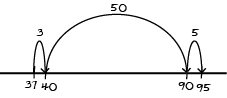the model uses a number line. an arched arrow labeled 3 points from 37 to 40. the next arched arrow, labeled 50, points from 40 to 90. the last arched arrow, labeled 5, points from 90 to 95.

2.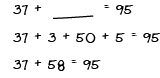the model uses three equations: 37 plus blank equals 95, 37 plus 3 plus 50 plus 5 equals 95, and 37 plus 58 equals 95.

3.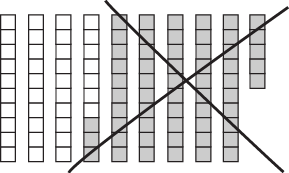the model uses columns of squares. there are 9 columns with 10 squares each and one column of 5 squares. the first 3 columns plus 7 squares of the next column are white, while the remaining squares are shaded. a large x has been drawn over the shaded squares.

4.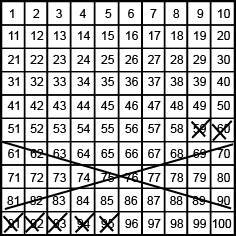the model uses a number grid. the first row is labeled 1 through 10, the second 11 through 20, and so forth to the bottom row, which is labeled 91 through 100. exes have been drawn through all the numbers from 59 to 95.

Answer and Rationale. Enter to expand or collapse. Answer expanded
Correct Response: A.
The student is using a counting on method to find the difference between 95 and 37. First the student counts up to the nearest multiple of ten from 37 to get to 40, then counts up a group of tens to get to 90. Lastly, the student counts up from 90 to 95. The number line model shows the same procedure, starting at 37 and jumping up to 40, then jumping forward by tens to 90, and last jumping up to 95. The arrows above the number line indicate how much each jump is worth and summing the arrow values gives the difference between 95 and 37, which is the number of pages the student has left to read. The number line shown in response A best models this strategy.

#### Objective 0003 Apply knowledge of properties of numbers and operations involving fractions and strategies for teaching these concepts to students.

5. Use the diagram below to answer the question that follows.The diagram shows a row of 6 squares. The first 4 squares are collectively labeled 1 whole.

A teacher uses a tape diagram during a lesson on fractions. If the labeled section on the diagram represents 1 whole, which of the following number bonds (addition facts) best matches the tape diagram?

Each response is a bond diagram showing a central number and a set of smaller numbers that add together to equal the central number.

1.the central number is 6 sixths. the smaller numbers are 4 sixths and 2 sixths.

2.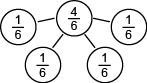the central number is 4 sixths. the smaller numbers are 1 sixth, 1 sixth, 1 sixth, and 1 sixth.

3.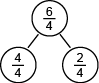the central number is 6 fourths. the smaller numbers are 4 fourths and 2 fourths.

4.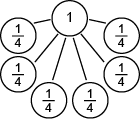the central number is 1. the smaller numbers are 1 fourth, 1 fourth, 1 fourth, 1 fourth, 1 fourth, and 1 fourth.

Answer and Rationale. Enter to expand or collapse. Answer expanded
Correct Response: C.
In the tape diagram shown, the whole is labeled as four sections of the tape, so each section is one-fourth. The entire tape diagram is made up of 6 of those sections,  four fourths  and  two fourths  resulting in the improper fraction  six fourths.  A bond diagram is a representation of the part-part-whole relationship of a number showing how smaller numbers make up a larger number. The bond diagram in response C models the fraction  six fourths  as the sum of  four fourths  and ,  two fourths  which matches the tape diagram.

#### Objective 0003 Apply knowledge of properties of numbers and operations involving fractions and strategies for teaching these concepts to students.

6. Use the student work below to answer the question that follows.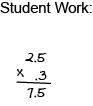A student multiplies two decimals as shown. Which of the following approaches would most likely help the student gain understanding of where to correctly position the decimal point when finding the product of two decimals?

1. writing out step-by-step instructions for multiplying decimals
2. converting the decimals to their fraction equivalents and then multiplying the fractions
3. creating a word problem to model decimal multiplication
4. using a calculator to multiply pairs of decimals and recording all the results in a table
Answer and Rationale. Enter to expand or collapse. Answer expanded
Correct Response: B.
The student incorrectly placed the decimal point so that it lined up with the decimal points in the numbers used in the multiplication. Converting the decimals to their fraction equivalents would allow the student to get the correct result using prior knowledge of how to multiply fractions. The fraction product of 25 tenths times 3 tenths equals 75 hundredths allows the student to understand why the result should be the decimal value of 75 hundredths, or 0.75.

#### Objective 0004 Apply knowledge of operations and algebraic thinking and strategies for teaching these concepts to students.

7. A student needs to find the greatest common factor of 12 and 18 to solve a word problem. Which of the following models of student work shows a valid method for finding this greatest common factor?

1.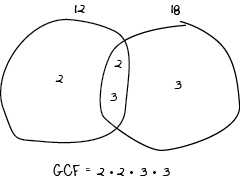the model is a venn diagram. the left circle is labeled 12, and the right circle is labeled 18. the left circle contains the number 2. the right circle contains the number 3. the intersection contains the numbers 2 and 3. beneath the diagram is written gcf equals 2 times 2 times 3 times 3.

2.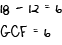the model shows the equation 18 minus 12 equals 6. beneath the equation is written gcf equals 2 times 3.

3.the model shows the factors of the 2 numbers. the first line is 12 equals 2 times 2 times 3. the second line is 18 equals 2 times 3 times 3. the 2 and the first 3 on the second line are vertically aligned with the second 2 and the 3 on the first line. vertical arrows are drawn through the aligned numbers, pointing down to the 2 and the 3 on the next line of text, which is gcf equals 2 times 3.

4.the model shows sets of multiples of the 2 numbers. the first line is 12, 24, 36, 48, dot, dot, dot. the second line is 18, 36, 54, 72, dot, dot, dot. the 36 in each line is circled, and a line connects the 2 circles. the final line is gcf equals 36.

Answer and Rationale. Enter to expand or collapse. Answer expanded
Correct Response: C.
The greatest common factor of a pair of numbers is the largest whole number value that is a factor of both numbers. One method of finding the greatest common factor is to do the prime factorization of both numbers and find the product of the prime factors that the two numbers have in common. The prime factorization of 12 is 2 times 2 times 3 and of 18 is 2 times 3 times 3. The prime factors that 12 and 18 have in common are 2 and 3 so the greatest common factor is 6.

#### Objective 0005 Apply knowledge of measurement and data and strategies for teaching these concepts to students.

8. Use the stem-and-leaf plot below to answer the question that follows.

Test Scores
Stem Leaf
2 2 5
3 cell intentionally left blank
4 3
5 1 8
6 5
7 3 5
8 2 6
9 4
10 0 0
Test Scores Key
Key
2/5 means 25

What is the median for the set of test scores shown?

1. 65
2. 68
3. 73
4. 78
Answer and Rationale. Enter to expand or collapse. Answer expanded
Correct Response: C.
To find the median of a data set, locate the value in the middle of the data set when all the values are arranged in numerical order. This stem and leaf plot has tens digits as the stems in the left hand column and ones digits as the leaves in the right hand column, as indicated by the key at the bottom. In a stem and leaf plot the values are already ordered. There are 13 test scores shown in this stem and leaf plot, so the middle value will be in the seventh position. The seventh value in the list is 73.

#### Objective 0005 Apply knowledge of measurement and data and strategies for teaching these concepts to students.

9. Use the diagram below to answer the question that follows.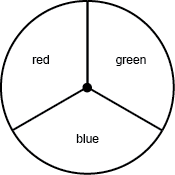The diagram is a spinner divided into three equal parts, labeled red, green, and blue.

A spinner is divided into thirds and each third is shaded a different color, as shown in the diagram. If the radius of the spinner is 6 inches, what is the perimeter of the green section of the spinner?

1. 16 pi
2. 22 pi
3. 4 pi plus 12
4. 12 pi plus 12
Answer and Rationale. Enter to expand or collapse. Answer expanded
Correct Response: C.
The green section of the spinner has three edges, the curved edge that is one third the circumference of the circle and two straight edges that are each equal to the radius of the circle. To find the circumference of a circle, multiply the diameter of the circle by pi. This spinner has diameter of 12 inches so the circumference of this spinner is 12 pi. One third of that is 12 pi over 3 equals 4 pi. The radius of the spinner is 6 inches so the perimeter of the green section is 4 pi plus 6 plus 6 equals 4 pi plus 12

#### Objective 0006 Apply knowledge of geometry and strategies for teaching geometry concepts to students.

10. Use the diagram below to answer the question that follows.The diagram shows 4 lines that intersect to form a 4 sided interior shape. From top to bottom, one line has points A, B, C, and D. From top to bottom, the opposite line has points E, F, G, and H. A third line passes through points B and F. The fourth line passes through points C and G.

The diagram shows a sketch of the relative positions of four lines on a plane. If angle FBC is congruent to angle DCG, then which of the following statements must be true?

1. Line B C is parallel to line F G.
2. Line B F is perpendicular to line C B.
3. Line C G is parallel to line B F.
4. Line F G is perpendicular to line C G.
Answer and Rationale. Enter to expand or collapse. Answer expanded
Correct Response: C.
Corresponding angles are formed when a transversal passes through two lines. The angles that are in the same position in terms of the transversal are called corresponding angles. Two lines are parallel if the corresponding angles formed by the transversal passing through them are congruent. Angles F B C and D C G are corresponding angles for the two lines C G and B F with transversal A D. Line C G is parallel to line B F.